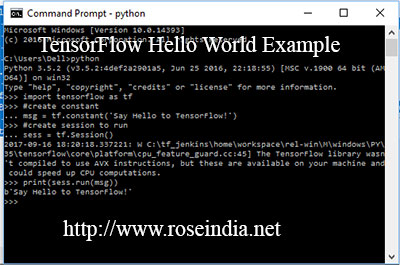# Hello World Program in TensorFlow

In the last section we have installed TensorFlow on Windows 10 computer, now in this session we will make our first Hello World TensorFlow example.## Writing and running Hello World Program in TensorFlow

After installation of Python and TensorFlow on Windows computer we will proceed with the programming series and first create famous Hello World program. We will write and run TensorFlow Hello World program.

TensorFlow is machine learning and distributed statistical computing framework which utilizes the power of modern CPU, GPU and TPU for running machine learning programs. TensorFlow is open source and famous deep machine learning framework used for developing large scale deep learning system.

In in this series of FensorFlow tutorials we will first make our Hello World program and run it through python interactive session.

If you have not installed Python and TensorFlow then check our tutorial on installing TensorFlow on windows 10 and then come back to this tutorial.

Here is the simple program in TensorFlow which prints "Say Hello to TensorFlow!", when executed.

Here is the example program:

```#TensorFlow Hello World example
#import tensorflow libraries
import tensorflow as tf
#create constant
msg = tf.constant('Say Hello to TensorFlow!')
#create session to run
sess = tf.Session()
print(sess.run(msg))
```

We are first importing TensorFlow library with following code:

import tensorflow as tf

Then creating a constant with the following code:

msg = tf.constant('Say Hello to TensorFlow!')

Constants value cannot be modified once created.

Then creating an instance of TensorFlow session with following code:

sess = tf.Session()

The tf.Session() is a session management class in TensorFlow which is responsible for running the TensorFlow operations. To run the TensorFlow operations we will use the method sess.run() as shown below:

print(sess.run(msg))

Above command will run the operations and print "Say Hello to TensorFlow!" on the screen as shown below:

```Microsoft Windows [Version 10.0.14393]

C:\Users\Dell>python
Python 3.5.2 (v3.5.2:4def2a2901a5, Jun 25 2016, 22:18:55) [MSC v.1900 64 bit (AMD64)] on win32
>>> import tensorflow as tf
>>> #create constant
... msg = tf.constant('Say Hello to TensorFlow!')
>>> #create session to run
... sess = tf.Session()
2017-09-16 18:20:18.337221: W C:\tf_jenkins\home\workspace\rel-win\M\windows\PY\35\tensorflow\core\platform\cpu_feature_guard.cc:45] The TensorFlow library wasn't compiled to use AVX instructions, but these are available on your machine and could speed up CPU computations.
>>> print(sess.run(msg))
b'Say Hello to TensorFlow!'
>>>
```In this session you learned to write your first TensorFlow Example program. In the next session we will learn how to use variables, constants in TensorFlow.